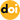### Direct Current Control Method Based On One Cycle Controller for Double-Frequency Buck Converters

Vol. 12, No. 3, pp. 410-417, May  201210.6113/JPE.2012.12.3.410

###### Abstract

In this paper, a direct current control method based on a one-cycle controller (DCOCC) for double frequency buck converters (DF buck) is proposed. This control method can make the average current through the high frequency and low frequency inductors of a DF buck converter equal. This is similar to the average current control method. However, the design of the loop compensator is much easier when compared with the average current control. Since the average current though the high frequency and low frequency inductors is equivalent, the current stress of the high frequency switches and the switch losses are minimized. Therefore, the efficiency of the DF buck converter is improved. Firstly, the operation principle of DCOCC is described, then the small signal models of a one cycle controller and a DF buck converter are presented based on the state space average method. Eventually, a system block diagram of the DCOCC controlled DF buck is established and the compensator is designed. Finally, simulation and experiment results are given to verify the correction of the theory analysis.

###### Statistics
Show / Hide Statistics

Cumulative Counts from September 30th, 2019
Multiple requests among the same browser session are counted as one view. If you mouse over a chart, the values of data points will be shown.